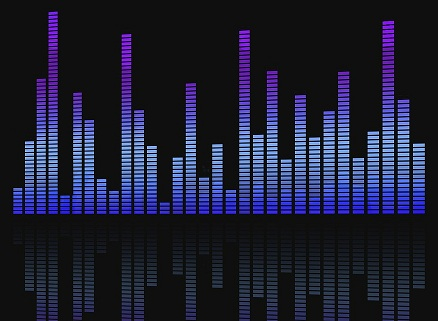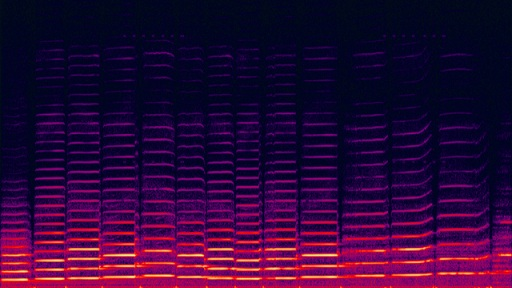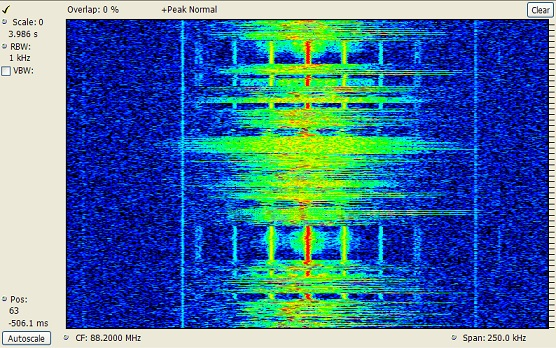# Difference Between Bandwidth and Frequency

## Main Difference – Bandwidth vs. Frequency

The terms bandwidth and frequency can have different meanings depending on the context. Here, we explore these terms with regards to their usage in the field of signal processing. The main difference between bandwidth and frequency is that frequency refers to the number of times that a component of a signal oscillates per second, whereas bandwidth refers to the range of frequencies that can be contained within a signal.

## What is Frequency

Data is often communicated using signals, and signals are made of waves. The frequency of a signal refers to the number of times the signal oscillates per second, and it is usually measured in hertz (Hz). In the case of sound, frequency is a measure of the sound’s pitch: High-frequency sounds make high-pitched (“treble”) sounds while low-frequency sounds make low-pitched (“bass”) sounds.

A signal can be considered to be formed by the addition of many waves with different frequencies, so a signal can be broken down into a power spectrum which gives how much power is carried by each of the component frequencies in a signal. “Equalizers” displayed on music-playing software are a simplified version of a power spectrum. It shows at what levels the different frequencies are present in the music being played at a given time:An “equalizer” display in a music player shows at what levels the different frequencies of sounds are present in a wave at any given time.

frequency spectrogram can be produced by using frequency and time as the x and y axes on the graph, and by using a colour scale to represent  the power of a particular frequency at a given time. For instance, the frequency spectrogram for a violin being played is shown below:Frequency spectrogram for a violin being played.

In the above diagram, the horizontal axis represents time while the vertical axis represents frequency. The 14 vertical “columns” indicate that 14 notes were played during the time. The small horizontal bars represent the harmonic frequencies making up each note.

## What is Bandwidth

Bandwidth refers to the range of component frequencies that is contained in a signal. If the minimum and maximum components of frequencies that occur in a modulated signal are$f_{min}$ and$f_{max}$, then the bandwidth is given by$f_{max}-f_{min}$.

When the bandwidth is larger, a larger number of frequencies can be represented by a signal. For instance, AM radio signals which have a bandwidth of 9-10 kHz fail to transmit higher frequencies of sound that FM radios, having bandwidths of 100-200 kHz, can transmit without a problem.

The diagram below shows the frequency spectrogram for an FM radio signal.On this graph, the horizontal axis represents the frequency while the vertical axis represents time. As you can see, there is a range of frequencies that the signal carries. This range is the bandwidth of this signal.

## Difference Between Bandwidth and Frequency

### Definition:

Frequency is the number of times that a wave oscillates per second. Since a signal can be made of many different waves acting as components, we can use the term frequency to refer to the number of times that a given component oscillates per second.

The term bandwidth refers to the total range of component frequencies that can be accommodated in a signal.

### Relationship with Pitch:

In terms of sound, frequency determines the pitch of the sound.

Bandwidth determines the range of pitch that can be encoded in a signal.

Image Courtesy:

“equalizer-eq-sound-level-digital-255396” by bykst (Own work) [CC0 1.0], via Pixabay (Modified)

“A spectrogram of a violin waveform…” by Antilived (Created by User:Omegatron using Adobe Audition) [CC BY-SA 3.0], via Wikimedia Commons

“Spectrogram of an FM-Radio transmission from germany…” by Casandro~commonswiki (Own work) [CC BY-SA 3.0], via Wikimedia Commons